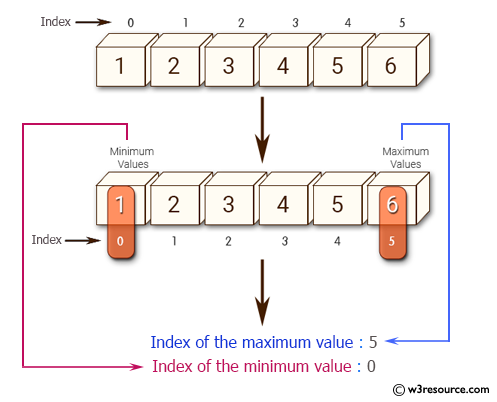﻿ NumPy: Find the indices of the maximum and minimum values along the given axis of an array - w3resource# NumPy: Find the indices of the maximum and minimum values along the given axis of an array

## NumPy: Array Object Exercise-27 with Solution

Write a NumPy program to find the indices of the maximum and minimum values along the given axis of an array.

Pictorial Presentation:Sample Solution:-

Python Code:

``````import numpy as np
x = np.array([1, 2, 3, 4, 5, 6])
print("Original array: ",x)
print("Maximum Values: ",np.argmax(x))
print("Minimum Values: ",np.argmin(x))
```
```

Sample Output:

```Original array:  [1 2 3 4 5 6]
Maximum Values:  5
Minimum Values:  0
```

Python Code Editor:

Have another way to solve this solution? Contribute your code (and comments) through Disqus.

What is the difficulty level of this exercise?

Test your Python skills with w3resource's quiz

﻿

## Python: Tips of the Day

Set comprehension:

```>>> m = {x ** 2 for x in range(5)}
>>> m
{0, 1, 4, 9, 16}
```